机器学习算法基础(1)：特征工程，文本特征抽取&tfidf方法1411-李同学

, ,

数据集的构成

mysql：

• 性能瓶颈，读取速度慢

• 格式不符合机械学习要求的数据格式

pandas：读取工具

kaggle

• 大数据竞赛平台

• 80 万科学家

• 真实数据

• 数据量巨大

UCI

• 收录了360个数据集

• 数据量几十万

• 覆盖各个领域

SK-LEARN

• 数据量较小

• 方便学习

常用数据集数据的结构组成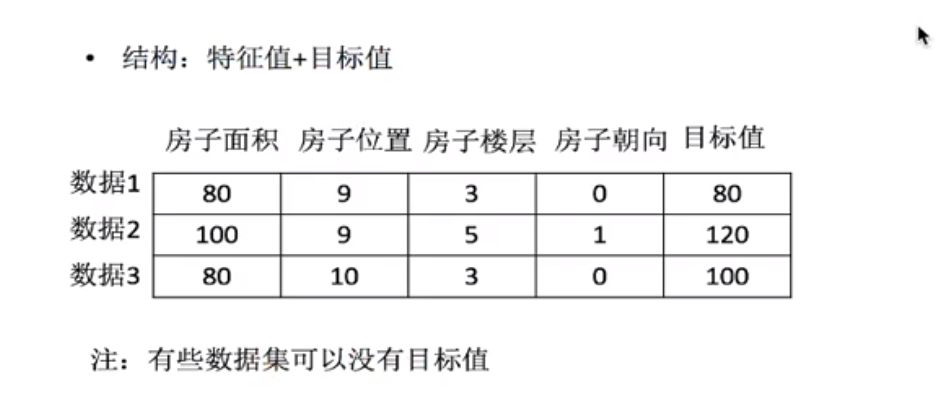数据对于特征的处理

pandas：一个数据读取非常方便以及基本的处理格式的工具

• 缺失值

• 数据转换

• 重复值 （机器学习中不需要进行去重）

sklearn：对于特征的处理提供了强大的接口

python语言的机器学习工具

• 数据的特征抽取

• 数据的特征预处理

• 数据的降维

字典特征数据抽取

sklearn特征抽取API

• 字典特征抽取
sklearn.feature_extraction.Dictvectorizer

Dictvectorizer语法

DictVectorizer.fit_transform(x)

流程

• 实例化DictVectorizer

• 输入数据并转换 fit_transform()

实例

from sklearn.feature_extraction import DictVectorizer

def dictvec():
#字典数据抽取
#实例化
dict=DictVectorizer(sparse=False)

#调用fit_transform,字典储存在列表中
data=dict.fit_transform([{'city': '北京','temperature': 100}
, {'city': '上海','temperature':60}, {'city': '深圳','temperature': 30}])

print(dict.get_feature_names())
print(dict.inverse_transform(data))
print(data)

if __name__=="__main__":
dictvec()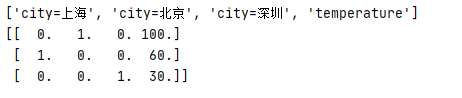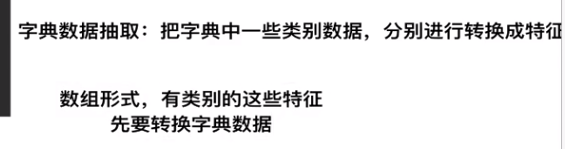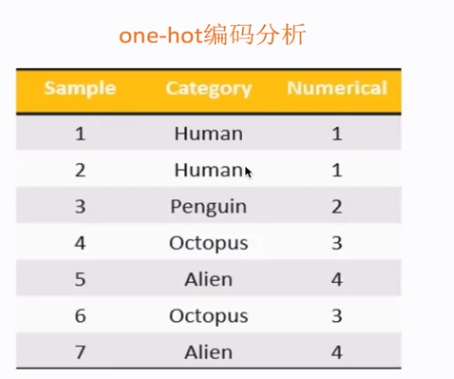文本特征抽取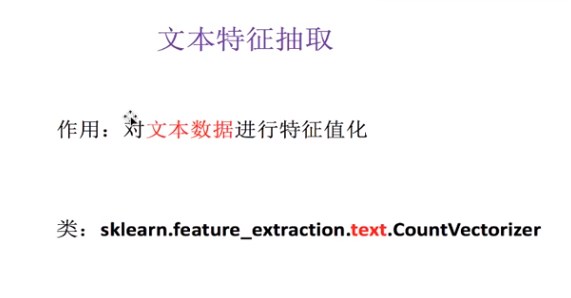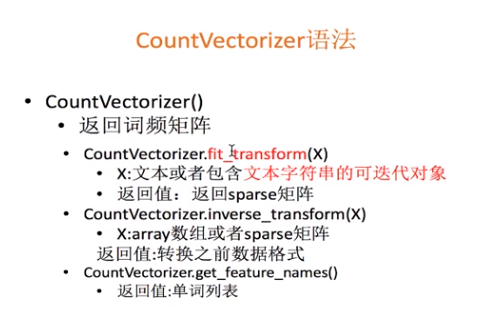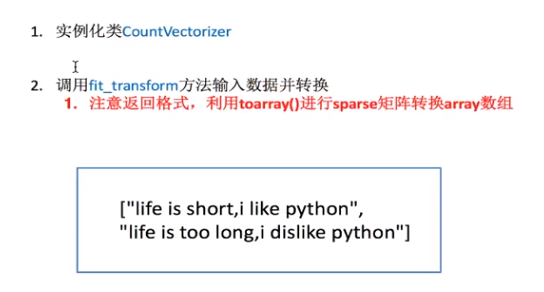实例

from sklearn.feature_extraction import DictVectorizer
from sklearn.feature_extraction.text import CountVectorizer
def countvec():
#对文本进行特征值化
CV=CountVectorizer()
data=CV.fit_transform(["life is short,i like python","life is too long,i dislike python"])
print(CV.get_feature_names()) #统计所有文章当中所有的词，重复的只看做一次 词的列表
print(data.toarray())   #对每篇文章，在词的列表里面进行统计每个词出现的次数

if __name__=="__main__":
countvec()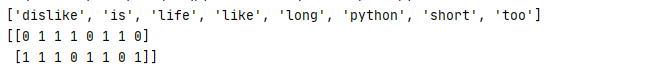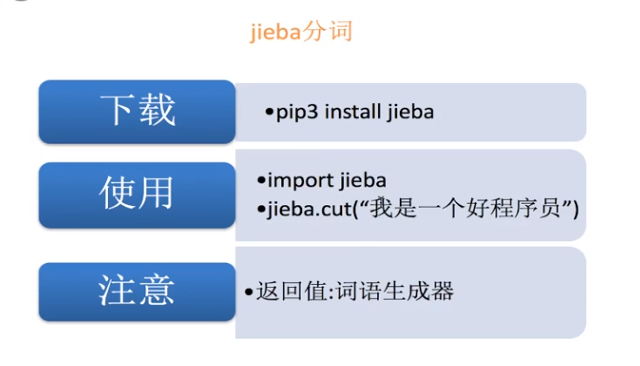对文本特征抽取分词并抽取特征值

from sklearn.feature_extraction import DictVectorizer
from sklearn.feature_extraction.text import CountVectorizer
import jieba
def cutword():
con1 = jieba.cut("今天很残酷，明天更残酷，后天很美好，但绝对大部分是死在明天晚上，所以每个人不要放弃今天。")

con2 = jieba.cut("我们看到的从很远星系来的光是在几百万年之前发出的，这样当我们看到宇宙时，我们是在看它的过去。")

con3 = jieba.cut("如果只用一种方式了解某样事物，你就不会真正了解它。了解事物真正含义的秘密取决于如何将其与我们所了解的事物相联系。")
#转换成列表
content1=list(con1)

content2=list(con2)

content3=list(con3)

#把列表换换成字符串
c1=" ".join(content1)
c2 = " ".join(content2)
c3 = " ".join(content3)
return c1, c2, c3

def hanzi():
#中文特征值化
c1,c2,c3=cutword()
print(c1,c2,c3)

CV = CountVectorizer()

data = CV.fit_transform([c1,c2,c3])  # 需要对中文进行分词处理

print(CV.get_feature_names())  # 统计所有文章当中所有的词，重复的只看做一次 词的列表

print(data.toarray())  # 对每篇文章，在词的列表里面进行统计每个词出现的次数

if __name__=="__main__":
hanzi()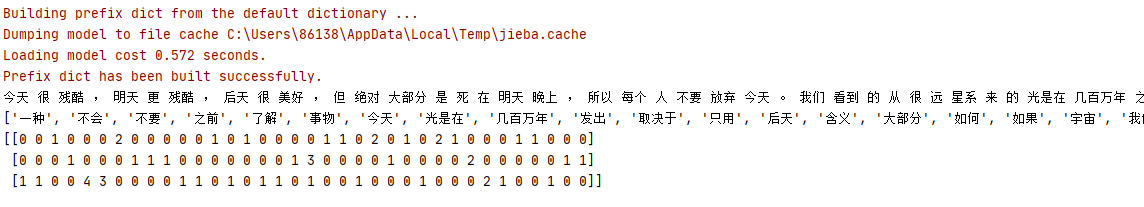tf_ idf分析

tf: term frequency(词的频率) 出现的次数

idf: 逆文档频率 inverse document frequency 公式：log(总文档数量/该词出现的文档数量)
log() 输入的数值越小，结果越小。

tf*idf 重要性 用以评估一个词对于一个文件集或一个语料库中的其中一份文件的重要程度。

sklearn.feature_extraction.text.TfidVectorizer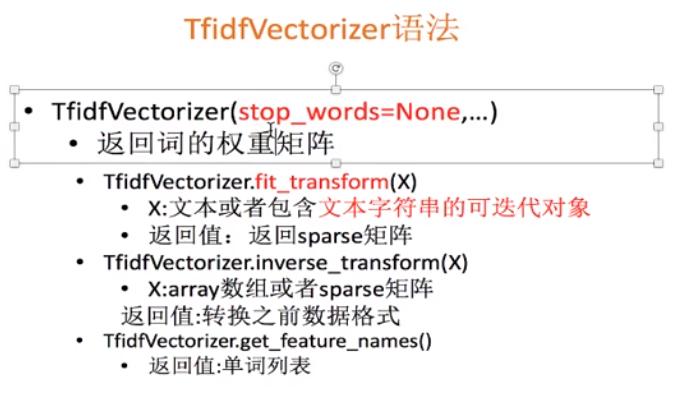from sklearn.feature_extraction import DictVectorizer
from sklearn.feature_extraction.text import CountVectorizer,TfidfVectorizer
import jieba
def tfidfvec():
#中文特征值化
c1,c2,c3=cutword()
print(c1,c2,c3)

tf = TfidfVectorizer()

data = tf.fit_transform([c1,c2,c3])  # 需要对中文进行分词处理

print(tf.get_feature_names())  # 统计所有文章当中所有的词，重复的只看做一次 词的列表

print(data.toarray())  # 对每篇文章，在词的列表里面进行统计每个词出现的次数

if __name__=="__main__":
tfidfvec()

评论 抢沙发觉得文章有用就打赏一下文章作者

支付宝扫一扫打赏微信扫一扫打赏Vieu3.3主题Q Q 登 录